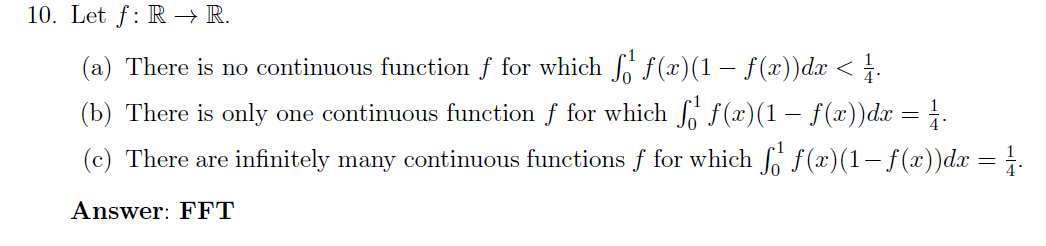# CMI integration problem

Home Forums Math Olympiad, I.S.I., C.M.I. Entrance CMI integration problem

Viewing 4 posts - 1 through 4 (of 4 total)
• Author
Posts
• #60258please give a correct explanation for this problem. it is obtained from cmi BSc math entrance examination 2019 problem number 10.

#60488

Will get back to you within 24 hours

#60719

take $f(x)=1$ then $f(x)$ is continuous and the integral becomes $0$ which is less than $\frac14$, so the first option is not valid.

We can see that for $f(x)=\frac12$ (constant function $\Rightarrow$ continuous) value of the integration is $\frac14$.

Now what do you think ? Are there infinite values so that the value of the integral is $\frac14$

#60990

So have you tried it?

3rd statement is not true :

$f(x)(1-f(x))=f(x)-f(x)^2$

$=\frac14 -(\frac14 -2.\frac12.f(x)+f(x)^2)$

$=\frac14-(\frac12-f(x)^2)$

Now let $g(x)=(1-f(x))^2\geq 0,$ then $g$ is positive and continuous :

then $\int_0^1 g(x)=0$ if and only if $g(x)=0$.

Since $g$ is continuous and $g(x)=0$ everywhere then$f(x)=\frac12$

Then $\int_0^1 f(x)(1-f(x)) \mathrm d x$

$=\int_0^1 \frac14 \mathrm d x -\int_0^1 (\frac12 – f(x))^2\mathrm d x$

$=\frac14-\int_0^1 (\frac12 – f(x))^2\mathrm d x$

$=\frac14$ if and only if $f(x)=\frac12$

Viewing 4 posts - 1 through 4 (of 4 total)
• You must be logged in to reply to this topic.